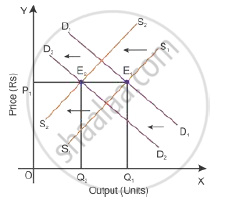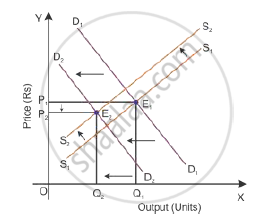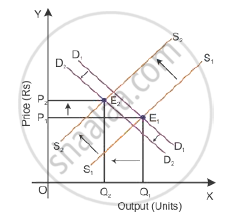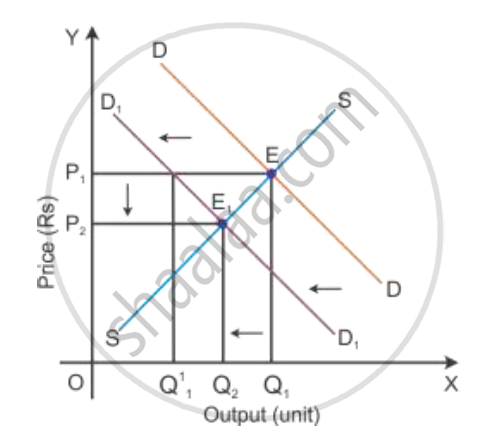# Market for a Good is in Equilibrium. There is Simultaneous "Decrease" Both in Demand and Supply of the Good. Explain Its Effect on Market Price - Economics

#### Questions

Market for a good is in equilibrium. There is simultaneous "decrease" both in demand and supply of the good. Explain its effect on market price

A market for a good is in equilibrium. The demand for the good 'decreases'. Explain the chain of effects of this change

#### Solution 1

When there is simultaneous decrease in demand and supply, the equilibrium point of price of output will depend on the basis of proportion of changes in demand and supply. Let us understand the effects through the following conditions.
i. If decrease in demand is equal to decrease in supply
When the demand decreases from D1D1 to D2D2 and the supply decreases from S1S1 to S2S2 in the same proportion, the new equilibrium price will be OP1 and the new equilibrium output will be OQ2. This new price and output intersect each other at Point E2 and will arrive at new equilibrium. As the decrease in demand is equal to the decrease in supply, the price remains the same and the quantity will decrease at the new equilibrium point.ii. If decrease in demand is more than decrease in supply
When the demand decreases, the demand curve will shift to D2D2 and the decrease in supply will shift the supply curve to S2S2. Here, the decrease in demand is more than the supply; hence, there will be a new equilibrium price and a new equilibrium output which intersects at Point E2. As the decrease in demand is more than the decrease in supply, the equilibrium price decreases and the equilibrium quantity level decreases at the new equilibrium point.iii. If decrease in demand is less than decrease in supply
When the demand decreases, the demand curve will shift to D2D2 and the decrease in supply will shift the supply curve to S2S2. Here, the decrease in demand is less than the supply; hence, there will be a new equilibrium price and a new equilibrium output which intersects at Point E2. As the decrease in demand is less than the decrease in supply, the equilibrium price increases and the equilibrium output decreases at the new equilibrium point.#### Solution 2

Consider DD1 to be the initial demand curve and SS to be the supply curve of the market. Market equilibrium is achieved at Point E, where the demand and supply curves intersect each other. Therefore, the equilibrium price is OP1, and the equilibrium quantity demanded is OQ1.

If there is fall in the demand, the demand curve will shift towards the left to DD1 and the supply curve SS will remain the same. This implies that at the initial price OP1, there is an excess supply of OQ1 to OQ11 units of output. Thereby the competition among producers will increase and they tend to reduce the price of the output to the OP2 level. Now, the new
market equilibrium will be at Point E1, where the new demand curve DD1 intersects the supply curve S

This clearly states that if the demand curve shifts towards the left, the price of the good tends to decrease with the decrease in the demand for a good. Thus, the direction of change in equilibrium price and the quantity are the same whenever there is a shift in the demand
curveIs there an error in this question or solution?